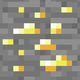# Unresolved Detail In Plotted Equations

Sometimes the calculator detects that an equation is too complicated to plot perfectly in a reasonable amount of time. When this happens, the equation is plotted at lower resolution.

Was this article helpful?
221 out of 246 found this helpful
Have more questions? Submit a request

#### 927 Comments

•Jaden Simis (Jadoof)

\cos\left(x\right)=\sin\left(xy\right)

•Sun Shengkun

\int_a^bf\left(x\right)dx=\sec y

it is lines but have unresolved details

•Sun Shengkun
•Sun Shengkun

\pi x+\cos\left(\frac{\pi x}{5}\right)\left(\sin\ xy\right)\cot\left(x\right)=y

hehe...

•GlitchedGraph666

\sum_{n_1=\sin\left(\sin\left(\sin\left(\sin\left(\sin\left(\sin\left(\sin\left(\sin\left(\sin\left(x!!!!!!!!!\right)\right)\right)\right)\right)\right)\right)\right)\right)}^{x^{x^{x^{x^{x^{\prod_{n_2=y^y}^{y^{2!^{x!!!!!!!!!!!!!!!!!!}}}n_2!!!!!!!!!!!!!!!!!!!^{n_2!!!!!!!}}+x}}}}}n_1^{x^{x^{x^{y^2}}}}+y^{y^{y^{y!!!!!!!!!!!!!!\cdot\prod_{n_{50}=2^{3^{4^{5^{6^{7^x}}}}}}^{\prod_{n_{100}=\prod_{n_{222}=666}^{x!!!!!!!!!!!!!}\left(n_{222}!^{n_{222}!^{n_{222}!}}\right)}^{\tan\left(y+\tan\left(y+\tan\left(y+\tan\left(y+\tan\left(y+\tan\left(y+\tan\left(y+\tan\left(y+\tan\left(y+\tan\left(y+\tan\left(y+x\right)\right)\right)\right)\right)\right)\right)\right)\right)\right)\right)}n_{100}!!!!!!!!}n_{50}!!!!!!!!!!}}}>x!!!!!!!!!!!!!!!!!!!!!!!!!!!!!!!!!!!!!!!!!!!!!!!!+y!!!!!!!!!!!!!!!!!!!!!!!!!!!!!!!!!!+\prod_{n_{732}=y!!!}^{x!!!}\sin\left(x!!!^{n_{732}!^{^{n_{732}!^{^{n_{732}!^{^{n_{732}!^{^{n_{732}!^{666}}}}}}}}}}\right)

•Charliedevelin

x!!!!!!!!!!!!!!!=y!!!!!!!!!!

•Andrew Carratu

and this:

\csc\left(\pi x+\cos\left(\frac{\pi x}{5}\right)\left(\sin\ xy\right)\cot\left(x\right)\right)=\sec\left(y\right)

•Orion D. Hunter

sin(x!)=sin(y!) and x!=y! actually behaves like x=y but it has a difference in desmos

•Orion D. Hunter

https://www.desmos.com/calculator/spjy78xgeb just, why, was, sin(x!!)=sin(y!!) not, plotted, like, X=Y

•Tanksear Industries
•Thecubicalguy1
•Joshua Lewinbl

mod(x,sin(xy)^2)=y

•168673
•Lie

This one is laggy so beware! \frac{x}{y}=\frac{3}{\sec\left(x^{5^y}\right)}

•Lie

This is not that detailed but I thought it looked SO COOL! \sqrt{x^2y^2}=\frac{9}{\sec\left(\pi x\right)}

•Lie

Put this in radian mode it looks cool: y=\frac{\cot\left(x\right)}{\tan\left(y\right)}

•Lie
•Orion D. Hunter
Edited by Orion D. Hunter
•Orion D. Hunter

some comments were not loading

•Lie

(but it has to be in radians degrees is too anticlimactic): x^y=9\sin\left(\cot\left(3x\right)\right)

•Filip Sedláček

2^x * 2^y=2^(x+y)

•D35M05BR34K4G3MA5T3R

How about some crazy art?  To see it, go to https://www.desmos.com/calculator/z5o7ikzpwu .

•Daemon Morrisoneagan

this never ends. no matter how far you zoom, the is an endless pattern. \log\left(\tan\left(\cos\left(\ln x^2\right)\right)\right)=\log\left(\cot\left(\sin\left(\ln y^2\right)\right)\right)

and this one i just can't explain.                                                                                        \log\left(\tan\left(\cos\left(\left(\ln x^2\right)!!\right)\right)\right)=\log\left(\cot\left(\sin\left(\left(\ln y^2\right)!!\right)\right)\right)

Edited by Daemon Morrisoneagan
•Tanksear Industries

\sin\left(x-\sqrt[x-y^{\frac{\sqrt{\sqrt{xy^{x^{\frac{y}{x^y}}}-x^{yx}}}}{\sqrt{x\sqrt{x\sqrt{y\sqrt{\sqrt{x}}}}}}}]{\sin\left(\sqrt{x-\frac{\sin\left(x\right)}{x-y}}\right)}\left|\sin\left(\sqrt{x}\right)\right|\right)\le\sin\left(\sqrt{\sqrt{x^y}\sqrt{y-x^{yxy}}}\right)

•Daemon Morrisoneagan
•ANDREW TUCKER

\tan\left(\frac{\sin\left(x+\tan\left(y\right)\right)}{y!-\sin\left(x\right)}\right)=\tan\left(\frac{\tan\left(y+\sin\left(x\right)\right)}{x!-\tan\left(y\right)}\right)

\sin\left(x!^{\log\left(y!\right)}\right)=\tan\left(y^{\log\left(x\right)}\right)

y=\tan\left(\frac{\sin\left(\tan\left(\frac{\tan\left(\frac{\sin\left(\frac{\sin\left(\tan\left(x\right)\right)}{\cos\left(\sin\left(y\right)\right)}\right)}{y-\tan\left(x\right)}\right)}{\tan\left(\tan\left(\frac{\tan\left(\tan\left(x\right)\right)}{y}\right)\right)}\right)\right)}{\tan\left(\tan\left(\tan\left(\frac{\tan\left(\sin\left(x\right)\right)}{y+\tan\left(x\right)}\right)\right)\right)}\right)

y=\tan\left(\frac{\tan\left(\frac{\sin\left(x+\tan\left(x\right)\right)}{y}\right)}{\sin\left(\tan\left(y\right)\right)}\right)

•Carson Perkins

y=tan(cos(x^5(y)))

•ANDREW TUCKER

\tan\left(\frac{\tan\left(\sin\left(x\right)\right)\sin\left(\log\left(y\right)\right)}{\sin\left(x!-\cos\left(y\right)\right)!}\right)=\cos\left(\sqrt{\sin\left(\frac{\tan\left(x+\sin\left(y!\right)\right)}{x\cos\left(y-\tan\left(\sin\left(x!\right)\right)\right)}\right)!}\right)

This one is a mess

https://www.desmos.com/calculator/hzyizn3wep

•Composer Machine

\tan\left(2\cdot\cos\left(x\right)\right)=\frac{\sin\left(y^2-x^2\right)}{\log\left(2\right)}

this makes an interesting design although it seems to form into a 'straighter line' as you zoom out on the left and right sides!

•Yang Pan

I was just bored
lol
https://www.desmos.com/calculator/uev2f3dwmw

This one does the opposite of what others do. The more you zoom out, the more it becomes focused. Until, it just stops loading.

Edited by Yang Pan
Article is closed for comments.